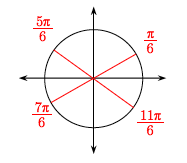### Home > CCA2 > Chapter Ch12 > Lesson 12.1.4 > Problem12-68

12-68.

Solve each equation below for $x$ if $-2π ≤ x ≤ 2π.$

1. $4\sin^2(x)=1$

$\sin^2(x)\ =\frac{1}{4}$

Divide both sides by $4$.

Take the square root of both sides to solve for sin$(x)$.

$\sin(x)\ =±\frac{1}{2}$

Find the corresponding angles on the unit circle.1. $3\tan^2(x)=1$

Follow the steps in part (a).